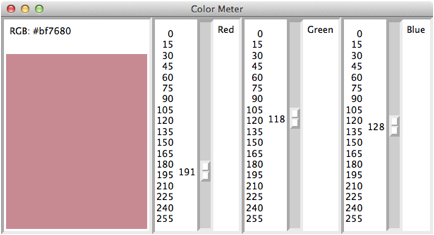A Simple Color Meter

A color meter program allows the user to view a color and its RGB value by dragging slider bars to indicate the red, green, and blue values of the color.  A color in this scheme has red, green, and blue components that range from 0 through 255, for a total of 224 possible colors.  An actual color value in Python is represented as a six-digit hexadecimal string, of the format xRRGGBB, where each pair of digits, RR, GG, and BB in the string correspond to the integer values of red, green, and blue, respectively.  Thus, the RGB integer values 10, 16, and 255 would produce the single RGB string "#0a10ff".

Here is the interface for the program (scaledemo2.py).Each of the three sliding scales has a vertical orientation, a label, and an interval from 0 to 255.  Note that the user has positioned the bars at 191 (red), 118 (green), and 128 (blue).  When any of the slider bars is adjusted, the program computes a six-digit hexadecimal string from these three values, displays this string in the upper left corner of the window, and repaints a canvas in the color that corresponds to this string.

Here is the code for the complete program (scaledemo2.py):

from breezypythongui import EasyFrame

from tkinter import VERTICAL

class ColorMeter(EasyFrame):

def __init__(self):

"""Sets up the window and widgets."""

EasyFrame.__init__(self, title = "Color Meter")

# Label to display the RGB value

self.rgbLabel = self.addLabel(text = "RGB : x000000",

row = 0, column = 0)

# Canvas to display the color

self.canvas = self.addCanvas(row = 1, column = 0)

self.canvas["bg"] = "black"

# Sliding scale for red

row = 0, column = 1,

orient = VERTICAL,

from_ = 0, to = 255,

length = 300,

tickinterval = 15,

command = self.setColor)

# Sliding scale for green

row = 0, column = 2,

orient = VERTICAL,

from_ = 0, to = 255,

length = 300,

tickinterval = 15,

command = self.setColor)

# Sliding scale for blue

row = 0, column = 3,

orient = VERTICAL,

from_ = 0, to = 255,

length = 300,

tickinterval = 15,

command = self.setColor)

# Event handler for the three sliding scales

def setColor(self, value):

"""Gets the RGB values from the scales,

converts them to hex, and builds a six-digit

hex string to update the view."""

red = hex(self.redScale.get())[2:]

green = hex(self.greenScale.get())[2:]

blue = hex(self.blueScale.get())[2:]

if len(red) == 1:

red = "0" + red

if len(green) == 1:

green = "0" + green

if len(blue) == 1:

blue = "0" + blue

color = "#" + red + green + blue

self.rgbLabel["text"] = "RGB: " + color

self.canvas["bg"] = color

# Instantiate and pop up the window.

ColorMeter().mainloop()

Note that each sliding scale object uses the same event handler method, setColor.  This is because the same action is performed when any slider bar is adjusted.  The setColor method ignores its value parameter, and instead uses the get method to obtain the RGB component from each of the sliding scale objects.